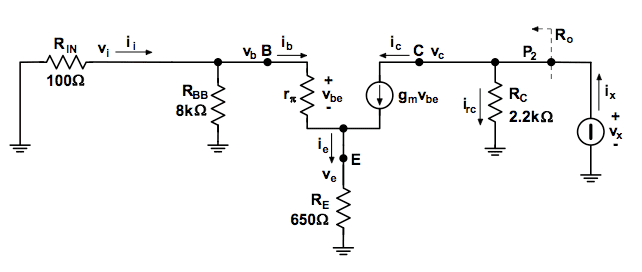# BJT small signal analysis: output resistance

eliotsbowe
Hello, I'm studying BJTs and I have a doubt about deriving the output resistance in the small signal equivalent circuit.

Let's consider the circuit below:To derive the output resistance, I'd write the following KCL:

$$i_x = i_{rc} + i_c$$

at this point, every example I've seen says $$R_o = R_c$$, that is: the collector current is zero.

May someone please explain me the reason for this statement?

yungman
It is my understanding if you want to find output impedance of the BJT, you don't have the Rc. Look at the collector curve in any transistor data sheet, they set up different Ib ( base current) and keep the base current constant. Then they vary the Vce and observe the change of the collector. Output resistance is:

$$\left |\frac {\partial I_c}{\partial V_{CE}}\right|_{ΔI_B=0}$$

This is done by curve tracer.

Your drawing miss a few things, namely output resistance $r_o$. And you don't want the 2.2K Rc there to drown out the $r_o$.

Your drawing miss a few things, namely output resistance $r_o$. And you don't want the 2.2K Rc there to drown out the $r_o$.

I'm pretty sure that his circuit is meant to be missing those things as it's a simplified analysis. It is common to ignore $r_o$ (or $h_{oe}$) when the external Rc dominates it anyway. The output resistance that the student wants here is meant to include Rc. Of course it is actually just Rc, since $r_o$ is being ignored.

$$i_x = i_{rc} + i_c$$

at this point, every example I've seen says $$R_o = R_c$$, that is: the collector current is zero.

May someone please explain me the reason for this statement?
That's because with all the other sources set to zero, $v_{be}$ is zero, therefore $v_{be}g_m$ is zero.

To think of it another way, it is not possible for the voltage $v_x$ on the output side to cause any voltage on the input side (that is, at the base emitter junction). Be aware that this is a somewhat simplified model that you are dealing with.

yungman
I'm pretty sure that his circuit is meant to be missing those things as it's a simplified analysis. It is common to ignore $r_o$ (or $h_{oe}$) when the external Rc dominates it anyway. The output resistance that the student wants here is meant to include Rc. Of course it is actually just Rc, since $r_o$ is being ignored.

Ha ha, just on the other post when I treat the question as simple transistor amp, turn out the OP did asked about early voltage and output resistance. I saw this post with the BJT model something like the Ebermal or something. I just look at it as real output resistance ro instead of just a simple circuit.

With the 2.2K, the output resistance is just 2.2K as ro is way into MΩ range.

Ratch
eliotsbowe,

To derive the output resistance, I'd write the following KCL:

ix=irc+ic

at this point, every example I've seen says Ro=Rc, that is: the collector current is zero.

May someone please explain me the reason for this statement?

Why do you say the collector current becomes zero when Rc=Ro? Rc is part of Ro.

If you want to see the full equation for the output resistance, look at the attachment. As successive terms are set to zero or infinity, the equation simplifies. Finally, as ro becomes infinity, the output impedance becomes simply Rc.

Ratch

#### Attachments

eliotsbowe
Thanks everyone for the replies.
First of all, I have to apologize because my question wasn't exhaustive and I forgot to mention that the Early effect was being ignored in my example.
That said, uart's answer is what I was looking for:

That's because with all the other sources set to zero, $v_{be}$ is zero, therefore $v_{be}g_m$ is zero.

To think of it another way, it is not possible for the voltage $v_x$ on the output side to cause any voltage on the input side (that is, at the base emitter junction). Be aware that this is a somewhat simplified model that you are dealing with.

I just have one last doubt.
In order to assume $v_{be}=0$, a zero base current $i_b$ should flow through $r_{\pi}$, right? But once the $i_x$ current has flown through Rc, couldn't it reach the left side of the circuit and "turn on" currents $i_b$ and $i_e$?

I just have one last doubt.
In order to assume $v_{be}=0$, a zero base current $i_b$ should flow through $r_{\pi}$, right? But once the $i_x$ current has flown through Rc, couldn't it reach the left side of the circuit and "turn on" currents $i_b$ and $i_e$?
Ok, say you apply KVL around the mesh on the left hand side (base emitter side) of your circuit. You should end up with something like:

$$[ R_B + r_\pi + (1 + g_m r_\pi) R_E] i_b = 0$$

Now since the part in the brackets is not zero, i_b therefore must be zero.

BTW. What I called R_B in the above equation is R_BB in parallel with R_in.

eliotsbowe
Ok, say you apply KVL around the mesh on the left hand side (base emitter side) of your circuit. You should end up with something like:

$$[ R_B + r_\pi + (1 + g_m r_\pi) R_E] i_b = 0$$

Now since the part in the brackets is not zero, i_b therefore must be zero.

BTW. What I called R_B in the above equation is R_BB in parallel with R_in.

That was helpful, thanks a lot!